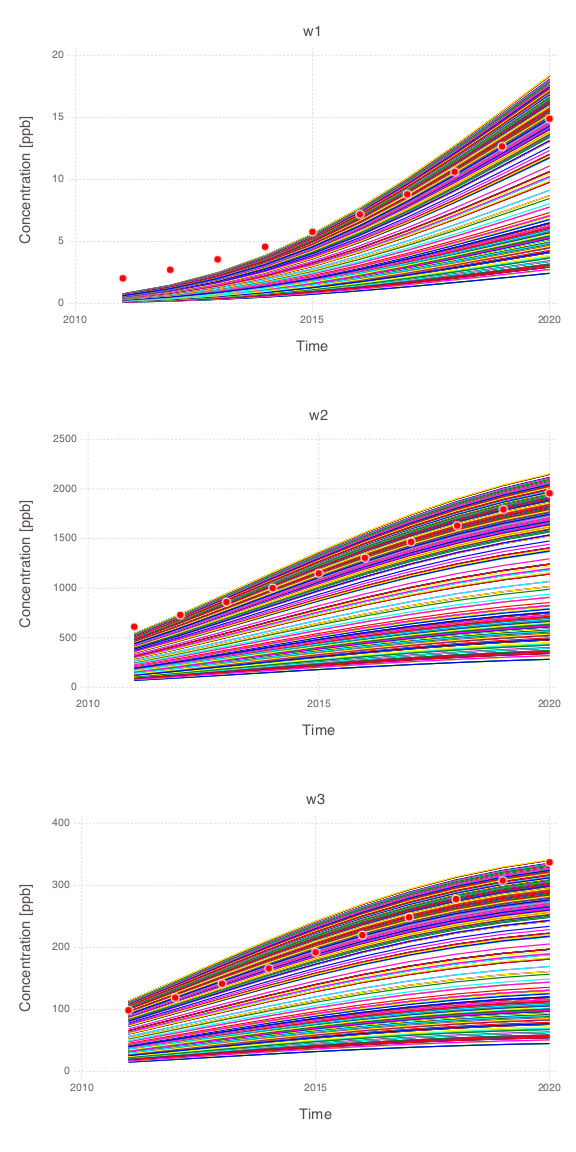Skip to content

# Uncertainty Quantification

## Bayesian Sampling

All the figures below are generated using examples/bayesian_sampling/bayesian_sampling.jl.

### Model setup• Contaminant source (orange rectangle)

• 3 monitoring wells

### Prior spaghetti plots

Spaghetti plots of 100 model runs representing the prior model prediction uncertainties at the 3 monitoring wells.

#### Joint spaghetti plots

All model parameters are changed simultaneously within their prior uncertainty ranges.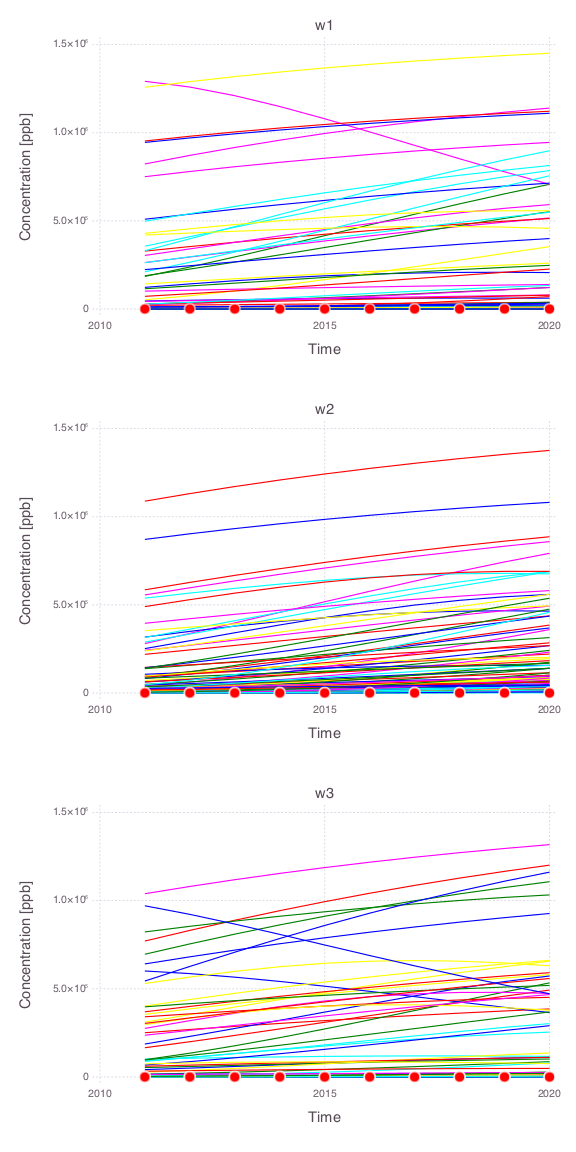#### Individual spaghetti plots

A single model parameter is changed at a time.

Source $x$ location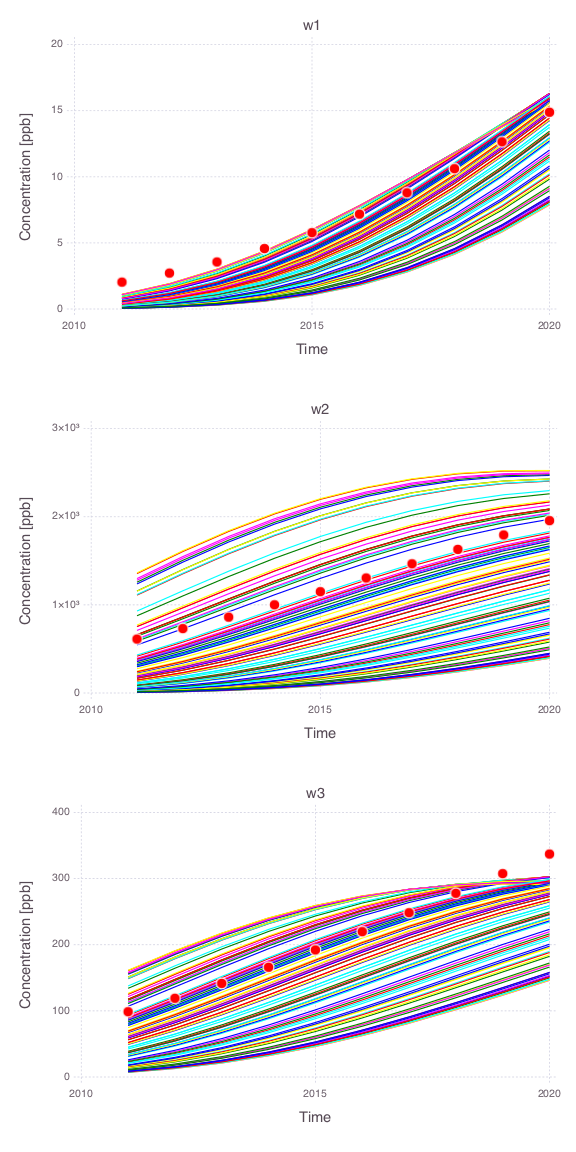Source $y$ location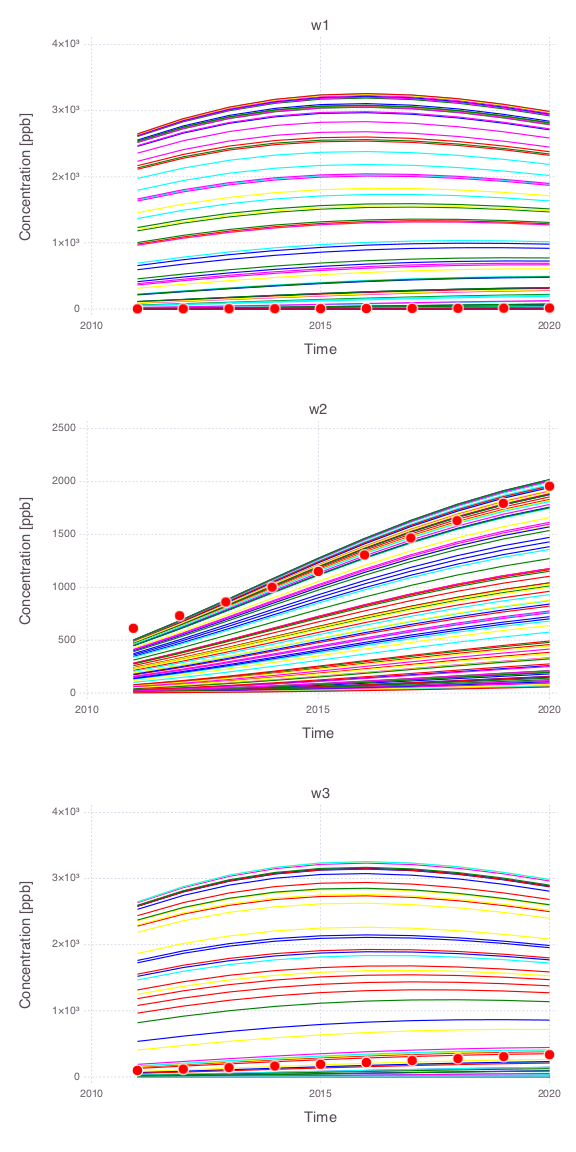Source size along $x$ axis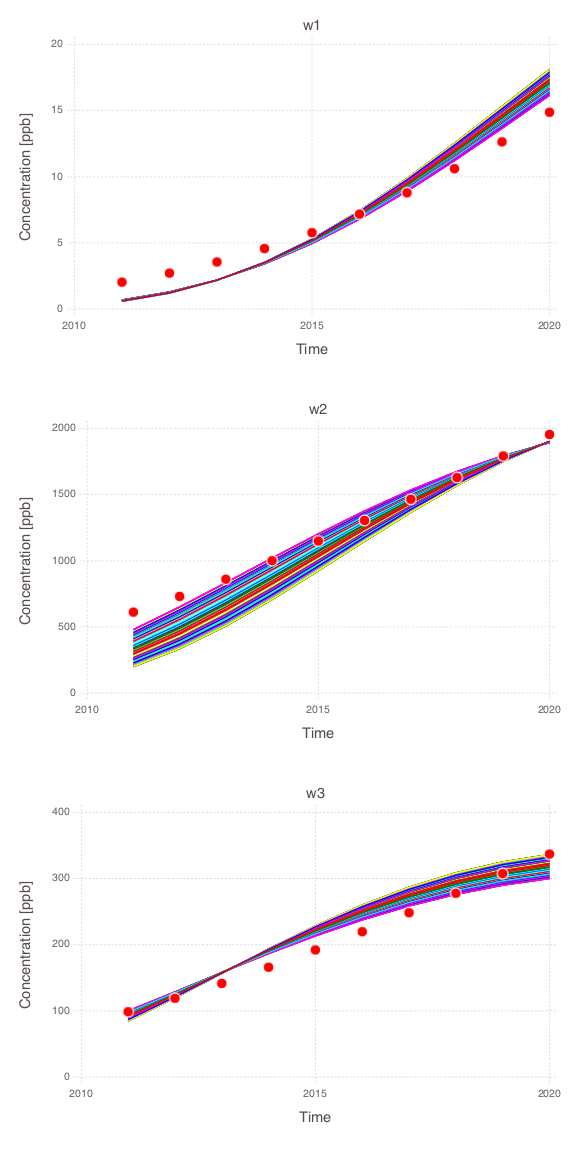Source size along $y$ axisSource release time $t_0$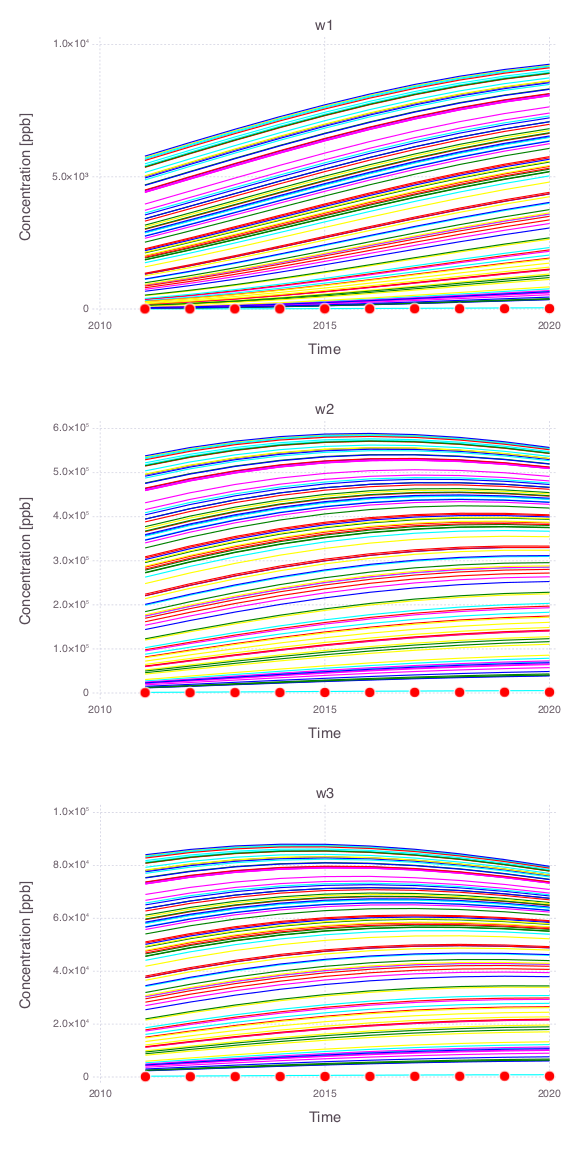Source termination time $t_1$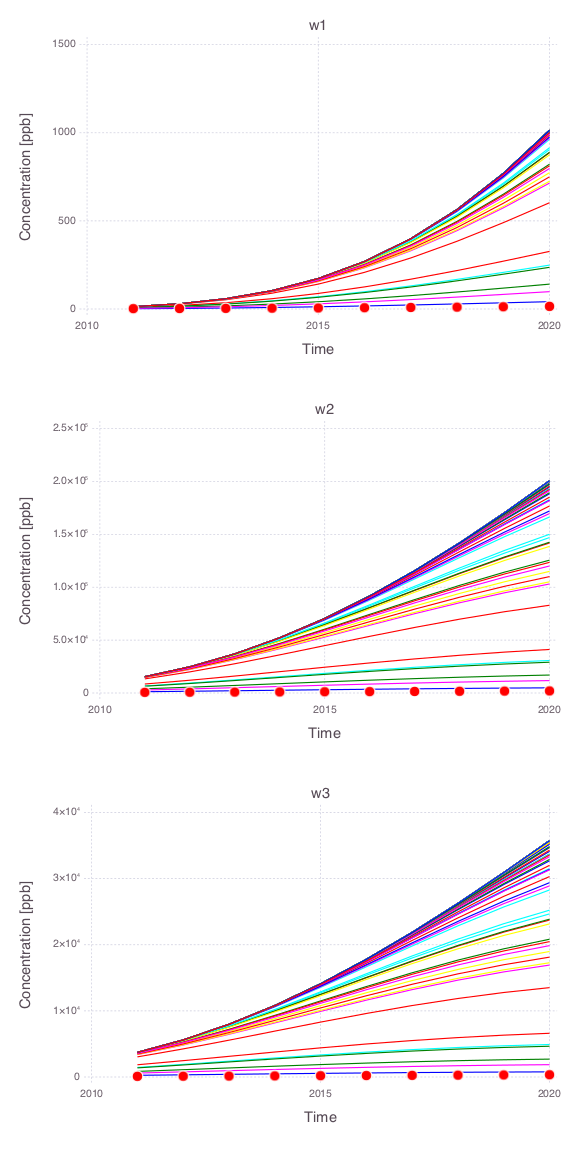### Model calibration match### Bayesian sampling results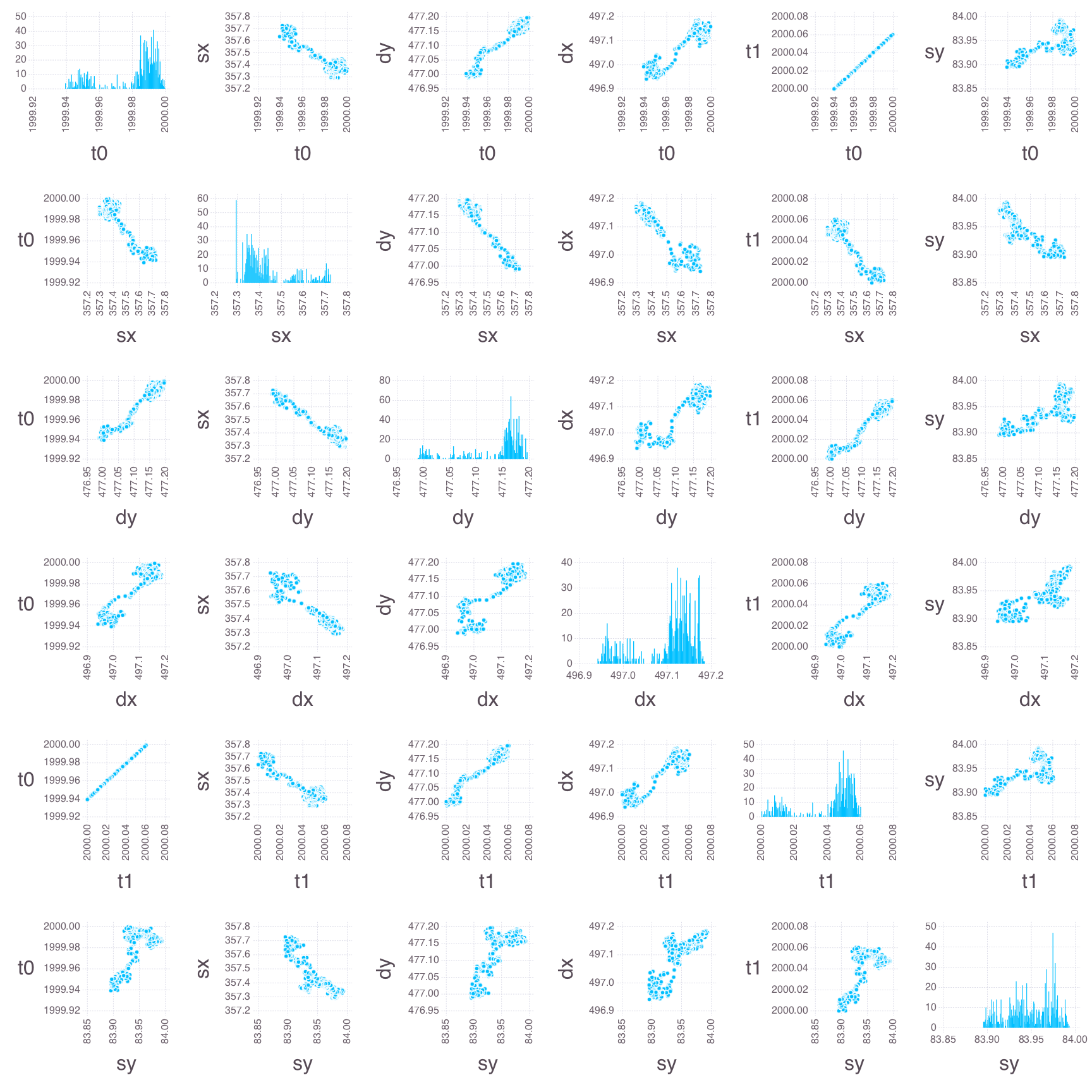### Posterior spaghetti plots

Spaghetti plots of 1000 model predictions representing the posterior model uncertainties at the 3 monitoring wells.

#### Joint spaghetti plots

All model parameters are changed simultaneously within their prior uncertainty ranges.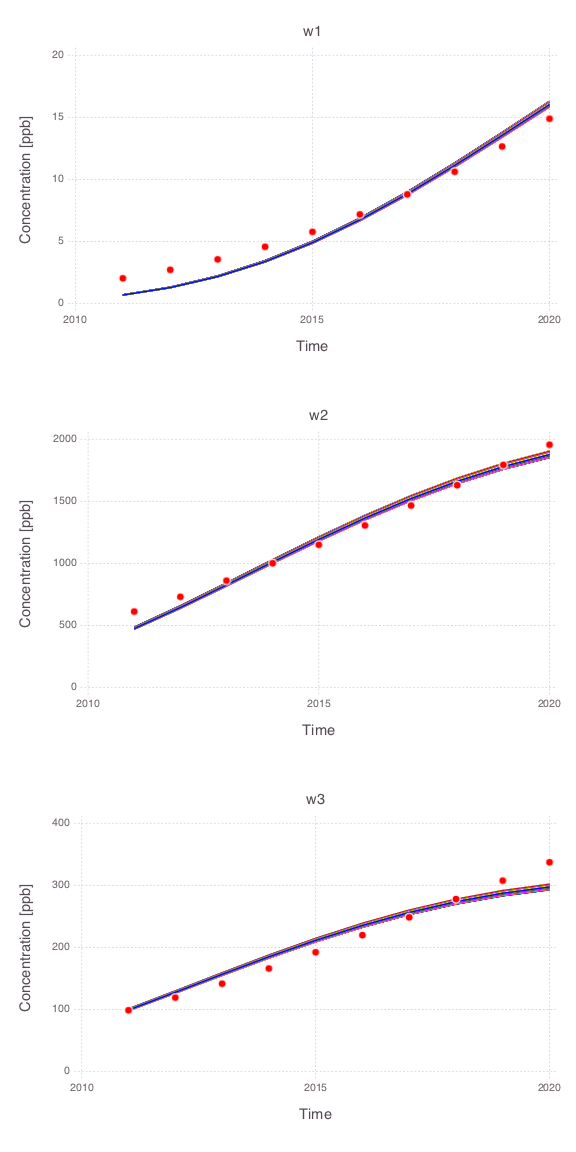#### Individual spaghetti plots

A single model parameter is changed at a time.

Note that only the posterior uncertainties in the source release time ($t_0$) and the source termination time ($t_1$) are producing large impact in the model predictions.

Source $x$ location (all the 1000 model predictions are overlapping)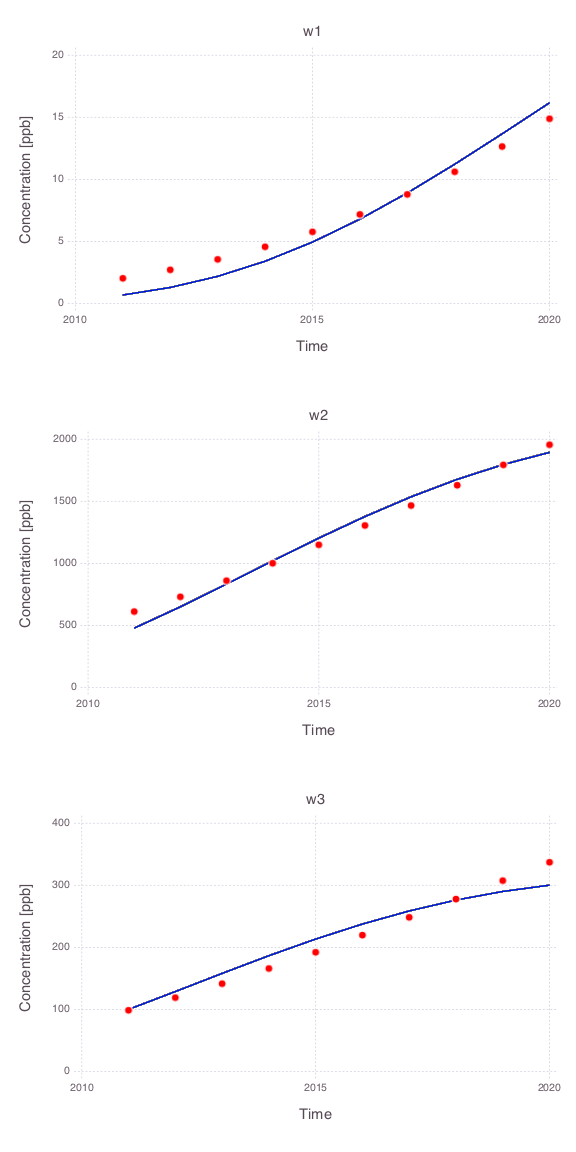Source $y$ location (all the 1000 model predictions are overlapping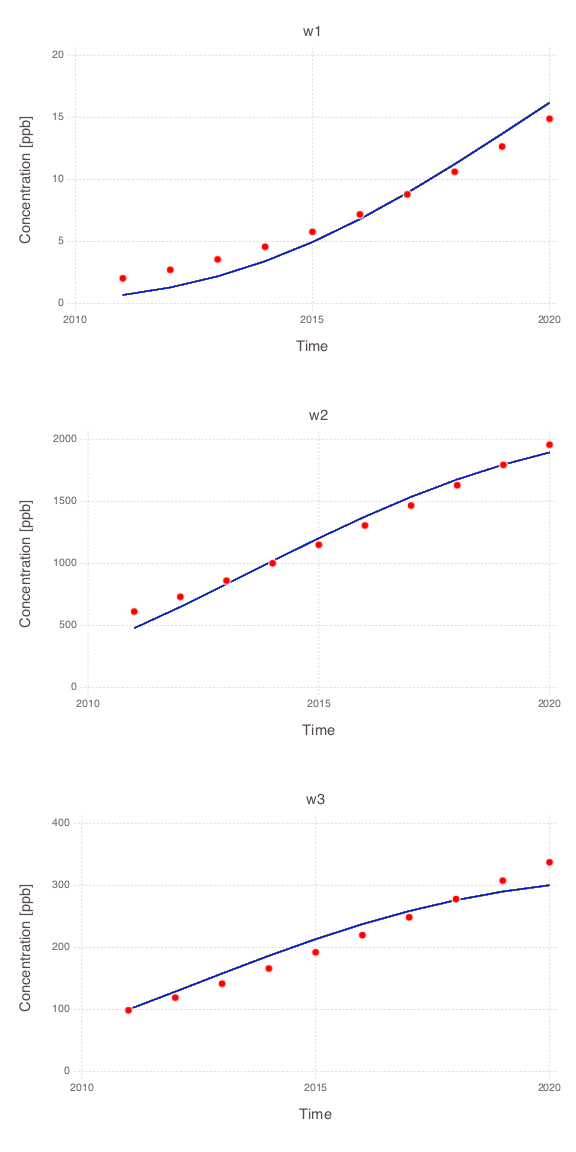Source size along $x$ axis (all the 1000 model predictions are overlapping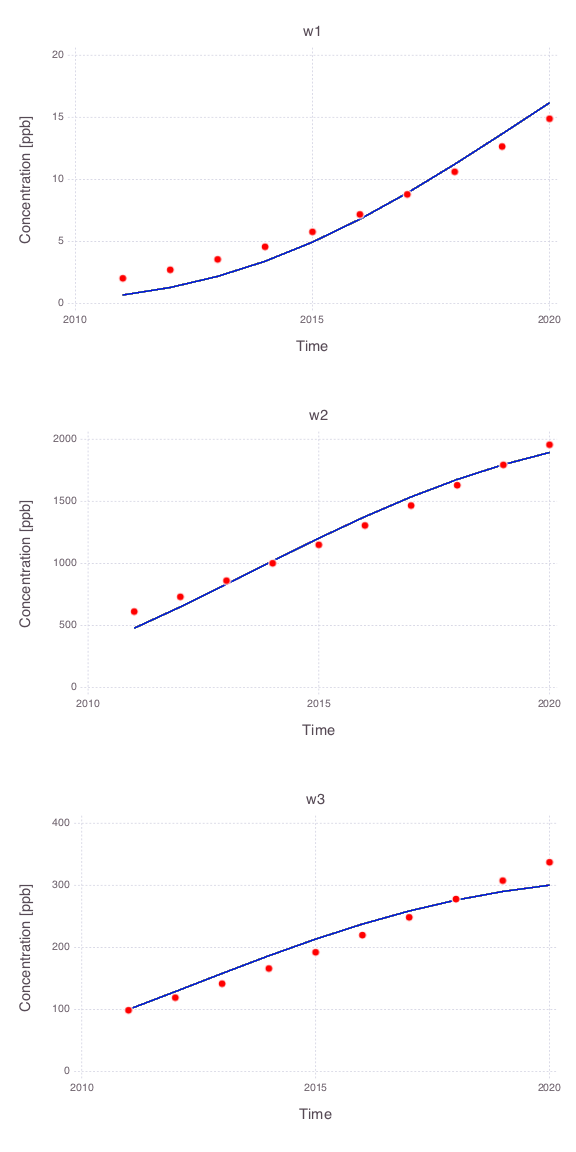Source size along $y$ axis (all the 1000 model predictions are overlappingSource release time $t_0$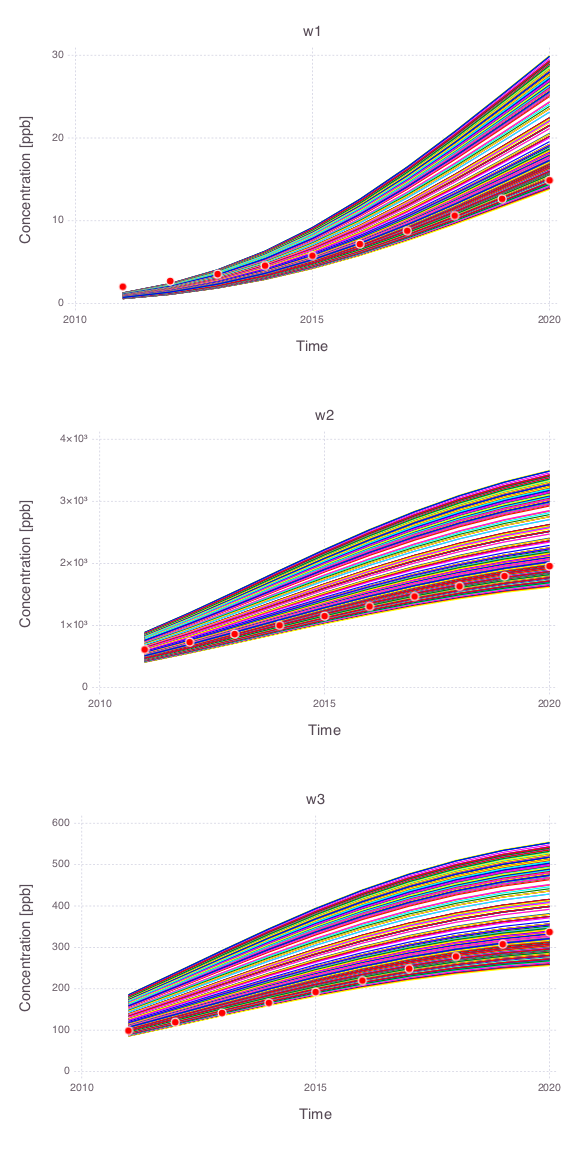Source termination time $t_1$